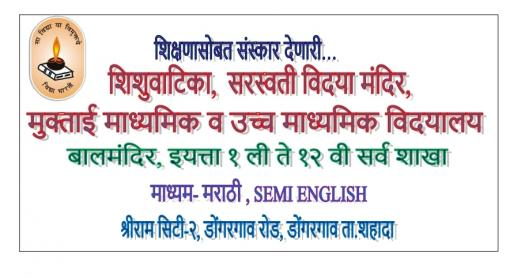# STD 1st Sub - English Mark20

Approved & Edited by ProProfs Editorial Team
The editorial team at ProProfs Quizzes consists of a select group of subject experts, trivia writers, and quiz masters who have authored over 10,000 quizzes taken by more than 100 million users. This team includes our in-house seasoned quiz moderators and subject matter experts. Our editorial experts, spread across the world, are rigorously trained using our comprehensive guidelines to ensure that you receive the highest quality quizzes.
| By Sandip Thakare
S
Sandip Thakare
Community Contributor
Quizzes Created: 9 | Total Attempts: 8,523
Questions: 16 | Attempts: 78SettingsQ. 1. Complete the words.

• 1.

### Wel___ome

• A.

B

• B.

C

• C.

D

B. C
Explanation
The missing letter in the word "Welcome" is "c". The pattern in the word suggests that each letter is followed by two line breaks, except for the missing letter "c". The letter "c" is followed by only one line break, making it the correct answer.

Rate this question:

• 2.

### H_____py

• A.

Ap

• B.

Cp

• C.

El

A. Ap
Explanation
The word "happy" is missing the letter "a" in the given options. Therefore, the correct answer is "ap" as it completes the word "happy".

Rate this question:

• 3.

### Ban__n__

• A.

A,b

• B.

A,a

• C.

D,a

B. A,a
Explanation
The correct answer is "a,a" because it follows the pattern of replacing the middle letter of the word with the letter "a". In the given word "Ban__n__", the middle letter "n" is replaced with "a", resulting in the word "Banaan". Therefore, the correct answer is "a,a".

Rate this question:

• 4.

### M_rni_g

• A.

A,n

• B.

C,n

• C.

O,n

C. O,n
Explanation
The correct answer is "on" because when we combine the letters from each row, we get the word "morning" with the missing letters being "o" and "n".

Rate this question:

• 5.

### M_ng_

• A.

A,o

• B.

D,a

• C.

A,q

A. A,o
Explanation
The word "M_ng_" can be completed by adding the letters "a" and "o" to form the word "Mango".

Rate this question:

• 6.

### Q.1.B) Odd man out. 1)Boy  2) Girl  3)Man   4)Bad

• A.

Boy

• B.

Girl

• C.

Man

• D.

Explanation
The word "Bad" is the odd man out because it does not belong to the category of gender or age, unlike the other options. "Boy," "Girl," and "Man" are all related to gender and age, while "Bad" is unrelated to these categories.

Rate this question:

• 7.

### 1)Dog  2)Cat  3)Tiger 4)Parrot

• A.

Dog

• B.

Cat

• C.

Tiger

• D.

Parrot

D. Parrot
Explanation
The given options are listed in a sequential order, starting with Dog, followed by Cat, then Tiger, and finally Parrot. The correct answer is Parrot because it is the last option listed in the sequence.

Rate this question:

• 8.

### 1)Mango  2)Banana  3) Apple  4)Ice -cream

• A.

Mango

• B.

Banana

• C.

Apple

• D.

Ice-cream

D. Ice-cream
Explanation
The given list consists of fruits (Mango, Banana, and Apple) and a dessert (Ice-cream). While the first three items are fruits, Ice-cream stands out as it is not a fruit but a sweet frozen treat. Therefore, the correct answer is Ice-cream.

Rate this question:

• 9.

### 1)Ox  2)Cow  3)Got 4)Tiger

• A.

Ox

• B.

Cow

• C.

Got

• D.

Tiger

D. Tiger
Explanation
The correct answer is Tiger because it is the only option that is not repeated in the given list. The other options, Ox, Cow, and Got, are all repeated twice.

Rate this question:

• 10.

### 1)Car   2) Bicycle   3) Tractor   4)Table

• A.

Car

• B.

Bicycle

• C.

Tractor

• D.

Table

D. Table
Explanation
The given options consist of different types of vehicles, except for the fourth option which is "Table". Therefore, the correct answer is "Table" as it does not belong to the category of vehicles.

Rate this question:

• 11.

### Q.2.B. Fill in the blank. A,B,C,__

• A.

D

• B.

E

• C.

G

A. D
Explanation
The given sequence follows a pattern where each letter is skipped in alphabetical order. Starting with A, the next letter is skipped (B), then the next letter is skipped (C), and so on. Therefore, the missing letter should be D.

Rate this question:

• 12.

• A.

Z

• B.

H

• C.

R

C. R
• 13.

### E,__G,H

• A.

C

• B.

F

• C.

D

B. F
Explanation
Based on the given arrangement, the letter 'F' is the only letter that is not connected to any other letter. It is not connected to any letter through a straight line or a diagonal line. Therefore, 'F' is the correct answer.

Rate this question:

• 14.

• A.

X

• B.

Y

• C.

W

A. X
• 15.

### S,T,U___

• A.

V

• B.

X

• C.

Q

A. V
Explanation
The pattern in the given sequence is that each letter is moving three positions forward in the alphabet. Starting with S, the next letter is V. Therefore, the correct answer is V.

Rate this question:

Related TopicsBack to top